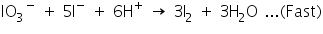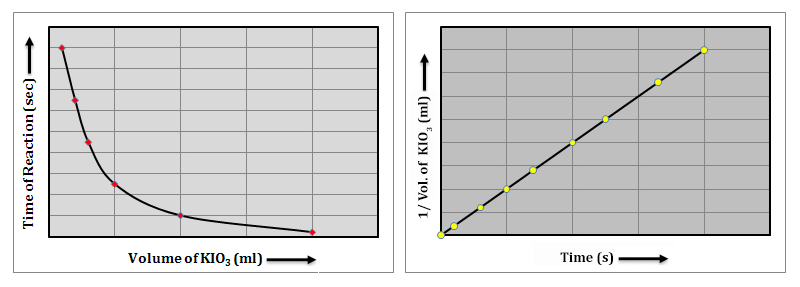Kinetics Study on the Reaction between Potassium iodate and Sodium Sulphite

# Objective

Our objective is to study the reaction rate of the reaction between potassium iodate (KIO3) and sodium sulphite (Na2SO3) using starch solution as indicator.

# The Theory

The rate of a chemical reaction may depend on the concentration of one or more reactants or it may be independent of the concentration of a given reactant. The rate dependance on the reactant concentration is expressed in an equation called the Rate Law.

For a general reaction of the type,, the rate law can be written as,Where, K is the rate constant, [A] and [B] are the concentration of the reactants, x and y are the exponents that define how the rate depends on the concentration of individual reactants. The exponents x and y are also referred to as the order of the reaction with respect to a particular reactant. The reaction order for each reactant in the rate law determines how the rate changes as the concentration of the reactant changes. If the order of a reactant is zero, the rate is independent of its concentration.

## Reaction between Potassium Iodate and Sodium Sulphite

The effect of concentration of the reactant on the rate of a chemical reaction can be studied by analysing the reaction between potassium iodate and sodium sulphite. In acidic medium, potassium iodate is reduced to iodide by sodium sulphite. The reaction takes place through the following steps.

Step I : Sulphite ions react with potassium iodate producing iodide ions.Step II: Iodide ions formed are oxidized to iodine by reaction with more iodate ions.Step III: Iodine formed in Step II reacts immediately with sulphite ions forming iodide ions.When sulphite ions are completely consumed, the liberated iodine would react with starch solution, to give a blue colour. So this reaction can be monitored by adding a known but limited volume of sodium sulphite solution and starch solution. This is an example of Iodine Clock Reaction, as the rate of reaction is estimated by the time taken for the appearance of blue colour. The faster the reaction, the shorter the time required for the blue colour to appear. Adding dilute solution of potassium iodate, decreases the concentration that decreases the rate of reaction. Conversely increasing the concentration of the reactant increases the rate of reaction.

The following graphs show that the rate of the reaction is directly depends on the concentration of potassium iodate.# Learning Outcomes

• Students understand the terms, rate law, iodine clock reaction etc.
• Strudents understand the chemistry behind the reaction between potassium iodate and sodium sulphite.
• Students acquire the skill to perform the experiment in the reals lab, once they understand the different steps.

Cite this Simulator: